Download Presentation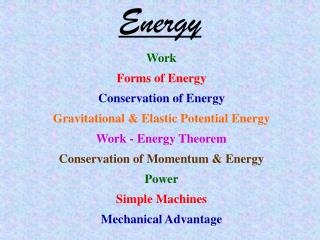Energy

# Energy - PowerPoint PPT Presentation

Energy. Work Forms of Energy Conservation of Energy Gravitational & Elastic Potential Energy Work - Energy Theorem Conservation of Momentum & Energy Power Simple Machines Mechanical Advantage. Work.I am the owner, or an agent authorized to act on behalf of the owner, of the copyrighted work described.
Download Presentation## Energy

An Image/Link below is provided (as is) to download presentation

Download Policy: Content on the Website is provided to you AS IS for your information and personal use and may not be sold / licensed / shared on other websites without getting consent from its author.While downloading, if for some reason you are not able to download a presentation, the publisher may have deleted the file from their server.

- - - - - - - - - - - - - - - - - - - - - - - - - - E N D - - - - - - - - - - - - - - - - - - - - - - - - - -
Presentation Transcript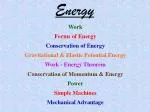Energy

Work

Forms of Energy

Conservation of Energy

Gravitational & ElasticPotential Energy

Work - Energy Theorem

Conservation of Momentum & Energy

Power

Simple Machines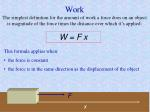Work

The simplest definition for the amount of work a force does on an object is magnitude of the force times the distance over which it’s applied:

W = Fx

• This formula applies when:
• the force is constant
• the force is in the same direction as the displacement of the object

F

x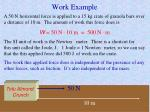Work Example

A 50 N horizontal force is applied to a 15 kg crate of granola bars over a distance of 10 m. The amount of work this force does is

W = 50 N·10 m = 500 N·m

The SI unit of work is the Newton · meter. There is a shortcut for this unit called the Joule, J. 1 Joule = 1 Newton·meter, so we can say that the this applied force did 500 J of work on the crate.

The work this applied force does is independent of the presence of any other forces, such as friction. It’s also independent of the mass.

Tofu Almond

Crunch

50 N

10 m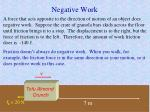Negative Work

A force that acts opposite to the direction of motion of an object does negative work. Suppose the crate of granola bars skids across the floor until friction brings it to a stop. The displacement is to the right, but the force of friction is to the left. Therefore, the amount of work friction does is -140 J.

Friction doesn’t always do negative work. When you walk, for example, the friction force is in the same direction as your motion, so it does positive work in this case.

v

Tofu Almond

Crunch

fk = 20 N

7 m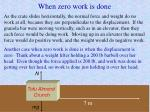When zero work is done

As the crate slides horizontally, the normal force and weight do no work at all, because they are perpendicular to the displacement. If the granola bar were moving vertically, such as in an elevator, then they each force would be doing work. Moving up in an elevator the normal force would do positive work, and the weight would do negative work.

Another case when zero work is done is when the displacement is zero. Think about a weight lifter holding a 200 lb barbell over her head. Even though the force applied is 200 lb, and work was done in getting over her head, no work is done just holding it over her head.

N

Tofu Almond

Crunch

7 m

mg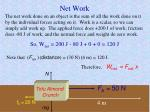Net Work

The net work done on an object is the sum of all the work done on it by the individual forces acting on it. Work is a scalar, so we can simply add work up. The applied force does +200 J of work; friction does -80 J of work; and the normal force and weight do zero work.

So, Wnet = 200 J - 80 J + 0 + 0 = 120 J

Note that (Fnet )(distance) = (30 N)(4 m) = 120 J.

Therefore,Wnet = Fnetx

N

Tofu Almond

Crunch

FA = 50 N

fk = 20 N

4 m

mg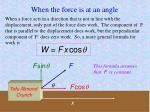When the force is at an angle

When a force acts in a direction that is not in line with the displacement, only part of the force does work. The component of F that is parallel to the displacement does work, but the perpendicular component of F does zero work. So, a more general formula for work is

W = Fxcos

Fsin

F

This formula assumes that F is constant.

Fcos

Tofu Almond

Crunch

x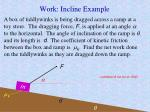Work: Incline Example

A box of tiddlywinks is being dragged across a ramp at a toy store. The dragging force, F, is applied at an angle to the horizontal. The angle of inclination of the ramp is, and its length is d.The coefficient of kinetic friction between the box and ramp is k. Find the net work done on the tiddlywinks as they are dragged down the ramp.

F

continued on next slide

m

k

d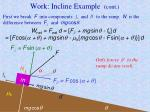Work: Incline Example (cont.)

First we break F into components  and //to the ramp. N is the difference between F and mgcos.

Wnet = Fnetd = [F// + mgsin - fk]d = [Fcos( +)+ mgsin - k{mgcos - Fsin( + )}]d

F =Fsin(+)

F

Only forces //to the ramp do any work.

N

fk

m

F// = Fcos(+)

mgsin

k

d

mgcos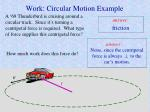Work: Circular Motion Example

A ‘69 Thunderbird is cruising around a circular track. Since it’s turning a centripetal force is required. What type of force supplies this centripetal force?

friction

None, since the centripetal force is always  to the car’s motion.

How much work does this force do?

v

rForms of Energy

When work is done on an object the amount of energy the object has as well as the types of energy it possesses could change. Here are some types of energy you should know:

• Kinetic energy
• Rotational Kinetic Energy
• Gravitational Potential Energy
• Elastic Potential Energy
• Chemical Potential Energy
• Mass itself
• Electrical energy
• Light
• Sound
• Other waves
• Thermal energyKinetic Energy

Kinetic energy is the energy of motion. By definition, kinetic energy is given by:

K = ½ mv2

• The equation shows that . . .
• . . . the more kinetic energy it’s got.
• the more mass a body has
• or the faster it’s moving

K is proportional to v2, so doubling the speed quadruples kinetic energy, and tripling the speed makes it nine times greater.Energy Units

The formula for kinetic energy, K = ½ mv2, shows that its units are:

kg·(m/s)2 = kg·m2/s2 = (kg·m/s2)m = N·m= J

So the SI unit for kinetic energy is the Joule, just as it is for work. The Joule is the SI unit for all types of energy.

One common non-SI unit for energy is the calorie. 1 cal = 4.186 J. A calorie is the amount of energy needed to raise the temperature of 1 gram of water 1 C.

A food calorie is really a kilocalorie. 1 Cal = 1000 cal = 4186 J.

Another common energy unit is the British thermal unit, BTU, which the energy needed to raise a pound of water 1 F. 1 BTU = 1055 J.Kinetic Energy Example

A 55 kg toy sailboat is cruising at 3 m/s. What is its kinetic energy?

This is a simple plug and chug problem:

K = 0.5 (55) (3)2 = 247.5 J

Note: Kinetic energy (along with every other type of energy) is a scalar, not a vector!Work - Energy Theorem:

The net work done on an object equals its change in kinetic energy.

Here’s a proof when Fnet is in line with the displacement, x. Recall that for uniform acceleration, average speed = v = ½(vf + v0).

Wnet = Fnetx = max = mx(v/t)

= m(x/t)v = mv(vf -v0)

= m[½(vf + v0)](vf -v0)

= ½m(vf2-v02) = ½mvf2-½mv02

= Kf - K0 = KWork - Energy Sample 1

Schmedrick takes his 1800 kg pet rhinoceros, Gertrude, ice skating on a frozen pond. While Gertrude is coasting past Schmedrick at 4 m/s, Schmedrick grabs on to her tail to hitch a ride. He holds on for 25 m. Because of friction between the ice and Schmedrick, Gertrude is slowed down. The force of friction is 170 N. Ignore the friction between Gertrude’s skates and the ice. How fast is she going when he lets go?

Friction, which does negative work here, is the net force, since weight and normal force cancel out. So, Wnet= -(170 N)(25 m)= -4250 J. By the work-energy theorem this is the change in her kinetic energy, meaning she loses this much energy. Thus,

-4250 J = K = ½ mvf2 - ½ mv02 = ½ m(vf2 - v02)

= ½ (1800 kg) [vf2 - (4 m/s)2]  vf = 3.358 m/s

You should redo this problem using the 2nd Law & kinematics and show that the answer is the same.Work-Energy Sample 2

A 62 pound upward force is applied to a 50 pound can of Spam. The Spam was originally at rest. How fast is it going if the upward force is applied for 20 feet?

Wnet = K

Fnetx = Kf - K0

(12 lb) (20 ft) = ½ mvf2 - 0

240 ft·lb = ½ (mg)vf2/g

240 ft·lb = ½ (50lb)vf2 /(32.2 ft/s2)

vf2 = 309.12 ft2/s2vf= 17.58 ft/s

multiply & divide by g

62lb

Spam

mg is the weight

9.8 m  32.2 ft

50lb

continued on next slideWork-Energy Sample 2 check

Let’s check our work with “old fashioned” methods:

vf2 - v02 = 2axvf2 = v02 + 2ax= 2ax

= 2(Fnet /m)x = 2Fnet g/(mg) ·x

= 2 (12lb)(32.2 ft/s2) / (50lb)·(20 ft)

= 309.12 ft2/s2

vf = 17.58 ft/s

This is the same answer we got using energy methods.Gravitational Potential Energy

Objects high above the ground have energy by virtue of their height. This is potential energy (the gravitational type). If allowed to fall, the energy of such an object can be converted into other forms like kinetic energy, heat, and sound. Gravitational potential energy is given by:

U = mgh

• The equation shows that . . .
• . . . the more gravitational potential energy it’s got.
• the more mass a body has
• or the stronger the gravitational field it’s in
• or the higher up it isSI Potential Energy Units

From the equation U = mghthe units of gravitational potential energy must be:

kg·(m/s2)·m = (kg·m/s2)·m = N·m= J

This shows the SI unit for potential energy is the Joule, as it is for work and all other types of energy.Reference point forUis arbitrary

Gravitational potential energy depends on an object’s height, but how is the height measured? It could be measured from the floor, from ground level, from sea level, etc. It doesn’t matter what we choose as a reference point (the place where the potential energy is zero) so long as we are consistent.

Example: A 190 kg mountain goat is perched precariously atop a 220 m mountain ledge. How much gravitational potential energy does it have?

U = mgh = (190) (9.8) (220) = 409640 J

This is how much energy the goat has with respect to the ground below. It would be different if we had chosen a different reference point.

continued on next slideReference point forU(cont.)

The amount of gravitation potential energy the mini-watermelon has depends on our reference point. It can be positive, negative, or zero.

D

6 m

Note: the weight of the object is given here, not the mass.

10 N

C

3 m

B

8 m

AWork and Potential Energy

If a force does work on an object but does not increase its kinetic energy, then that work is converted into some other form of energy, such as potential energy or heat. Suppose a 10 N upward force is applied to our mini-watermelon over a distance of 5 m. Since its weight is 10 N, the net force on it is zero, so there is no net work done on it. The work-energy theorem says that the melon undergoes no change in kinetic energy. However, it does gain gravitational potential energy in the amount of U = mgh = (10 N) (5 m) = 50 J. Notice that this is the same amount of work that the applied force does on it: W = Fd = (10 N) (5 m) = 50 J. This is an example of the conservation of energy.

FA = 10 N

10 N

mg = 10 NConservation of Energy

One of the most important principles in all of science is conservation of energy. It is also known as the first law of thermodynamics. It states that energy can change forms, but it cannot be created or destroyed. This means that all the energy in a system before some event must be accounted for afterwards.

before

m

For example, suppose a mass is dropped from some height. The gravitational potential energy it had originally is not destroyed. Rather it is converted into kinetic energy and heat. (The heat is generated due to friction with the air.) The initial total energy is given by E0 = U = mgh. The final total energy is given by Ef = K + heat = ½mv2 + heat. Conservation of energy demands that E0 = Ef.

Therefore, mgh = ½mv2 + heat.

heat

after

m

vConservation of Energy vs. Kinematics

Many problems that we’ve been solving with kinematics can be solved using energy methods. For many problems energy methods are easier, and for some it is the only possible way to solve them. Let’s do one both ways:

A 185 kg orangutan drops from a 7 m high branch in a rainforest in Indonesia. How fast is he moving when he hits the ground?

kinematics:

Conservation of energy:

E0 = Ef

mgh = ½mv2

2gh = v2

v = [2(9.8)(7)] ½ = 11.71 m/s

vf2 - v02 = 2ax

vf2 = 2(-9.8)(-7)

vf = 11.71 m/s

Note: the mass didn’t matter in either method. Also, we ignored air resistance in each, meaning a is a constant in the kinematics method and no heat is generated in the energy method.Waste Heat

The thermal energy that is converted from other forms due to friction, air resistance, drag, etc. is often referred to as “waste heat” because it represents energy “robbed from the system.” In real life some of the potential energy the orangutan had in the last example would have been converted to waste heat, making his fur and the surrounding air a tad bit hotter. This means that the ape has less kinetic energy upon impact than he had potential energy up in the tree. Air resistance robbed him of energy, but all the energy is still account for.

What happens to all his energy after he drops and is just standing still on the ground?(Now he has no kinetic or potential energy.)

It all ends up as waste heat. A small amount of energy is carried off as sound, but that eventually ends up as waste heat as well.Incline / friction example

A crate of Acme whoopy cushions is allowed to slide down a ramp from a warehouse into a semi delivery truck. Use energy methods to find its speed at the bottom of the ramp.

answer: The grav. potential energy at the top is partly converted kinetic energy. Friction turns the rest into waste heat. The work that friction does is negative, and the absolute value of it is the heat energy generated during the slide.

Acme

k= 0.21

continued on next slide

4 m

18 mIncline / friction example (cont.)

Let h = height of ramp;  = angle of inclination;d = length of ramp.

E0 = Ef U = | Wf | + K

mgh = fkd + ½ mv2 = (kmgcos)d + ½ mv2

gh = kg(18/d)d + ½ v2 = kg(18) + ½ v2

v = [2gh - 36 kg]½ = [19.6(4) - 36(0.21)(9.8)]½

v = 2.07 m/s

Note that we never actually had to calculate d or .

Acme

k= 0.21

4 m

18 mIncline / friction example II

Schmedrick shoves a 40 kg tub of crunchy peanut butter up a ramp. His pushing forces is 600 N and he pushes it 3 m up the ramp. When he stops pushing, the tub continues going up. What max height does it reach?answer: Let WS = the work done by Schmedrick, which is all converted to heat (by friction) and potential energy. Therefore, WS = | Wf | + U = fk(x+3) + mgh = (kmgcos16)(x+3)+mgh. Since sin16 = h/(x+3),x+3 = hcsc16.* This means WS= (kmgcos16)(hcsc16) + mgh. Factoring, we get WS= mgh[k cos16 (csc16) + 1]. Sincek= 0.19 and WS= (600 N)(3 m) = 1800 J, we solve forhand get 2.76 m.* csc = 1/sin

Peanut

Butter

sliding phase

pushing phase

x

h

Peanut

Butter

3 m

k = 0.19

16Elastic & Inelastic Collisions
• An elastic collision is one in which the total kinetic energy of colliding bodies is the same before and after, i.e., none of the original kinetic energy is converted to wasted heat.
• An inelastic collision is one in which at least some of the kinetic energy the bodies have before colliding is converted to waste heat.
• Apurelyinelastic collision occurs when two bodies stick together after colliding.
• In real life almost all collisions are inelastic, but sometimes they can be approximated as elastic for problem solving purposes.
• The collision of air molecules is truly elastic. (It doesn’t really make sense to say waste heat is generated since the motion of moleculesisthermal energy.)Elastic Collision

Since no waste heat is created in an elastic collision, we can write equations to conserve both momentum and energy. (In a closed system--meaning no external forces--momentum is conserved whether or not the collision is elastic.)

before:

after:

v1

v2

vA

vB

m1

m2

m1

m2

conservation of momentum:

m1v1 - m2v2 = -m1vA + m2vB

conservation of energy:

½m1v12 + ½m2v22 = ½m1vA2 + ½m2vB2

(Energy is a scalar, so there is no direction associated with it.)Elastic Collision Example

A 95 g rubber biscuit collides head on with an 18 g superball in an elastic collision. The initial speeds are given. Find the final speeds.

before:

after:

vB

vA

6 m/s

8 m/s

18 g

95 g

conservation of momentum:

(95g)(6m/s) - (18g)(8m/s) = -(95g)vA + (18g)vB

No conversion tokgneeded; grams cancel out.

426 = -95vA + 18vB

conservation of energy:

½(95g)(6m/s)2 + ½ (18g)(8m/s)2 = ½(95g)vA2 + ½(18g)vB2

continued on next slide

4572 = 95vA2 + 18vB2

cancel halves:Elastic Collision Example (cont.)

Both final speeds are unknown, but we have two equations, one from conserving momentum, and one from conserving energy:

momentum: 426 = -95vA + 18vB energy: 4572 = 95vA2 + 18vB2

If we solve the momentum equation forvBand substitute that into the energy equation, we get:

4572 = 95vA2 + 18[(426 +95vA)/18]2

Expanding, simplifying, and solving the quadratic gives us vA = -6 m/s or -1.54 m/s. Substituting each of these values into the momentum equation gives us the correspondingvB’s (in m/s):

{ vA = -6, vB = -8 } or{ vA = -1.54,vB = 15.54 }

continued on next slideAnalysis of Results

before:

after:

vB

vA

6 m/s

8 m/s

18 g

95 g

The interpretation of the negative signs in our answers is that we assumed the wrong direction in our after picture. Our first result tells us thatm1is moving to the right at 6 m/s and m2is moving at 8 m/s to the left. This means that the masses missed each other instead of colliding. (Note that when the miss each other both momentum and energy are conserved, and this result gives us confidence that our algebra is correct.) The second solution is the one we want. After the collisionm1is still moving to the right at 1.54 m/s, andm2rebounds to the right at 15.54 m/s.

{ vA = -6, vB = -8 } or{ vA = -1.54,vB = 15.54 }

miss collisionInelastic Collision Problem

Schmedrick decides to take up archery. He coerces his little brother Poindexter to stand 20 stand paces away with a kumquat on his head while Schmed takes aim at the fruit. The mass of the arrow is 0.7 kg, and when the bow is fully stretched, it is storing 285 J of elastic potential energy. (Things that can be stretched or compressed, like springs, can store this type of energy.) The kumquat’s mass is 0.3 kg. By the time the arrow hits the kumquat, friction and air resistance turn 4% of the energy it originally had into waste heat. Surprisingly, Schmedrick makes the shot and the arrow goes completely through the kumquat, exiting at 21 m/s. How fast is the kumquat moving now?

continued on next slideInelastic Collision (cont.)

First let’s figure out how fast the arrow is moving when it hits the fruit. 96% of its potential energy is turned to kinetic:

0.96(285) = ½ (0.7)v2 v = 27.9592 m/s

0.7 kg

0.3 kg

27.9592 m/s

vK

v = 0

21 m/s

before

after

Now we conserve momentum, but not kinetic energy, since this is not an elastic collision. This means that if we did not know the final speed of the arrow, we would not have enough information.

0.7(27.9592) = 0.3 vK + 0.7(21) vK = 16.2381 m/s

continued on next slideInelastic Collision (cont.)

How much more of the arrow’s original energy was lost while plowing its way through the kumquat?

0.7 kg

0.3 kg

27.9592 m/s

v = 0

16.2381 m/s

21 m/s

before

after

Before impact the total kinetic energy of the system is

K0= ½ (0.7)(27.9592)2 = 273.6 J

After impact the total kinetic energy of the system is

Kf= ½ (0.7)(21)2 + ½ (0.3)(16.2381)2 = 193.9 J

Therefore, 79.7 J of energy were converted into thermal energy. This shows that the collision was indeed inelastic.Thor

Elastic Collision in 2-D

The Norse god Thor is battling his archenemy--the evil giant Loki. Loki hurls a boulder at some helpless Scandinavian folk. Thor throws his magic hammer in order to deflect it and save the humans. Assuming

an elastic collision and that even the gods must obey the laws of physics, determine the rebound speed of the boulder and the final velocity of Thor’s hammer.

200 kg

105 kg

41

35

170 m/s

85 m/s

before

after

71

vB

vH

continued on next slideElastic Collision in 2-D (cont.)

200 kg

105 kg

41

35

170 m/s

85 m/s

before

after

71

vB

vH

horizontal momentum:

105(170)cos41 - 200(85)cos35 = -105vHcos + 200vBcos71

vertical momentum (down is +):

105(170)sin41 + 200(85)sin35 = 105vHsin + 200vBsin71

kinetic energy (after canceling the ½’s):

105(170)2 + 200(85)2 = 105vH2 + 200vB2

continued on next slideElastic Collision in 2-D (cont.)

71

vB

vH

The left side of each equation can be simplified, but we have a system of 3 equations with 3 unknowns with first degree, second degree and trigonometric terms. This requires a computer. With some help from Mathematica, we get vH= 94 m/s,  = -22.3º, and vB= 133.3 m/s. Since  is measured below the horizontal, the negative sign means the hammer bounced back up, which makes sense because Thor’s magic hammer always returns to him.

94 m/s

22.3º

71

133.3 m/sElastic Potential Energy

Things that can be stretched or compressed can store energy--elastic potential energy. Examples: a stretched rubber band; a compressed spring; a bent tree branch on a trebuchet catapult.

The elastic potential energy stored in a spring depends on the amount on stretch or compression and the spring constant. Recall, Hooke’s law: F = -kx, where: F is the force the spring exerts on whatever is stretching or compressing it; x is the amount of stretch or compression from the equilibrium point; and k is the spring constant. Like the force, the potential energy of a spring (or anything that obeys Hooke’s law) depends on k and x. It is given by:

U=½kx2

Note the similarity to the kinetic energy formula.Proof on next slide.U = ½ kx2 Derivation

As you stretch or compress a spring, the force you must apply varies. Let’s say you stretch it a distance x from equilibrium. In doing so, the force you apply ranges from zero (at the beginning) to kx (at the end). Since Hooke’s law is linear, the average force you applied is½ kx. Since this force is applied for a distance x, the work you do is½ kx2, and this is the energy now stored in the spring.Elastic Potential Energy Example

How much energy is stored in this spring? answer:

The energy stored is the same as the work done on the spring by whatever force stretched it out.

Since the force required grows as the spring stretches, we can’t just use W = Fd. To compute work directly would require calculus because of the changing force. However, we’ll use our new formula: U=½kx2= 0.5(800 N/m)(0.3 m)2 = 36 (N/m)·m2 = 36 N·m= 36 J

k = 8 N/cm

30 cm

Note that the units work out to energy units.Spring / projectile problem

After Moe hits Curly on the head with a hammer Curly retaliates by firing a dart at him with a suction cup tip from spring-loaded dart gun. The dart’s mass is 15 g and the spring constant of the spring in the gun is 22 N/cm. When loaded, the spring is compressed 3 cm. Curly fires the gun at an angle of 19° below the horizontal from up on a ladder. He misses Moe, but the dart hits Larry and sticks to his forehead 1.4 m below. What is the range of Curly’s dart?

Hints on next slide.Stooge problem hints

Summary of info:m = 15 g; k = 22 N/cm; x = 3 cm; y = -1.4 m;  = -19°

1. Calculate elastic potential energy:

2. Find kinetic energy of dart leaving gun:

3. Draw velocity vector and split into components.

Horizontal component:

Vertical component:

4. Use kinematics to find hang time:

5. Use d = vt to find range:

0.99 J

11.4891 m/s

10.8632 m/s

-3.7405 m/s

0.2751 s

2.99 mPower

Power is defined as the rate at which work is done. It can also refer to the rate at which energy is expended or absorbed. Mathematically, power is given by:

W

P =

t

Since work is force in the direction of motion times distance, we can write power as:

P = (Fxcos)/t = (Fcos)(x/t) = Fvcos.

F

Fsin

Fcos

x

mPower Example 1

1. Schmedrick decides to pump some iron. He lifts a 30 lb barbell over his head repeatedly: up and down 40 times in a minute and a half. With each lift he raises the barbell 65 cm. What is his power output? answer: Technically, the answer is zero, since each time Schmed lowers the bar the negative work he does negates the positive work he does in lifting it. Let’s calculate his power in lifting only: P = W/t = Fx/t. The force he applies is the weight of the barbell, and he completes 40 lifts. Since 1 kg weighs about 2.2 lb on Earth, we have:

P = 40(30 lb)(1 kg/2.2 lb)(9.8 m/s2)(0.65 m)/(90 s)

= 38.61 kg (m/s2)(m)/(s) = 38.61 N·m/s = 38.61 J/s.

This means Schmedrick, on average, does about 39 Joules of work each second. A Joules per second has a shortcut name--the Watt. Its symbol is W, and it is the SI unit for power.

continued on next slidePower Example 1 (cont.)

In actuality Schmedrick’s power output was greater than 38.61 W. This is because humans (and even machines) are not perfectly efficient. Schmed expended about 39 J of energy each second just in lifting the weights. This energy came from the chemical potential energy stored in his muscles. However, his muscles were not able to put all the energy into lifting. Some was wasted as heat. So, his body was really using up more than 39 J of energy each second.

If Schmedrick were 100% efficient when it comes to lifting, no waste heat would have been produced, but this is never the case in real life.Power Example 2

2. After pumping iron good, ole Schmed figures he ought to get in some aerobic exercise. He has too many allergies to run outside, so he decides to run stairs indoors. The flight of stairs has 80 steps, and the steps average 24 cm high. What is his power output running the flight once in 25 s? answer: Schmedrick weighs 105 lb (4.45 N/lb) = 467.25 N. (We could have converted to kilograms and multiplied by g and gotten the same weight in Newtons.) This time Schmed is lifting his own weight, so this is the force he must apply. The distance he applies this force is 80 (0.24 m) = 19.2 m. Thus his power output is:

(467.25 N)(19.2 m)/(25 s) = 358.85 W

Once again, Schmedrick’s actual rate of energy expenditure (power) would be greater than this since not all of his energy goes into lifting his body up the stairs. Waste heat, air resistance, etc. use some too.Light bulbs, Engines, & Power bills
• Light bulbs are rated by their power output. A 75 W incandescent bulb emits 75 J of energy each second. Much of this is heat. Fluorescent bulbs are much more efficient and produce the same amount of light at a much lower wattage.
• The power of an engine is typically measured in horsepower, a unit established by James Watt and based on the average power of a horse hauling coal. 1 hp = 33000 foot pounds per minute = 746 W. Note that in the English units we still have force times distance divided by time. A machine that applies 33000 pounds of force over a distance of one foot over a time period of one minute is operating at 1 hp.
• Electric companies charge customers based on how many kilowatt hours of energy used. It’s a unit of energy since it is power × time. 1 kW·h is the energy used by a 1000 W machine operating for one hour. How many Joules is it?

3.6 MJSimple Machines

Ordinary machines are typically complicated combinations of simple machines. There are six types of simple machines:

Simple Machine Example / description

• Lever
• Incline Plane
• Wedge
• Screw
• Pulley
• Wheel & Axle

crowbar

ramp

chisel, knife

drill bit, screw (combo of a wedge & incline plane)

wheel spins on its axle

door knob, tricycle wheel (wheel & axle spin together)Simple Machines: Force & Work

By definition a machine is an apparatus that changes the magnitude or direction of a force. Machines often make jobs easier for us by reducing the amount of force we must apply, e.g., pulling a nail out of a board requires much less force is a pry bar is used rather than pulling by hand. However, simple machines do not normally reduce the amount of work we do! The force we apply might be smaller, but we must apply that force over a greater distance.

A complex machine, like a helicopter, does allow a human to travel to the top of a mountain and do less work he they would by climbing, but this is because helicopters have an energy source of their own (gasoline). Riding a bike up a hill might require less human energy than walking, but it actually takes more energy to get both a bike and a human up to the top that the human alone. The bike simply helps us waste less energy so that we don’t produce as much waste heat.Force / Distance Tradeoff

Suppose a 300 lb crate of silly string has to be loaded onto a 1.3 m high silly string delivery truck. Too heavy to lift, a silly string truck loader uses a handy-dandy, frictionless, silly string loading ramp, which is at a 30º incline. With the ramp the worker only needs to apply a 150 lb force (since sin30º = ½). A little trig gives us the length of the ramp: 2.6 m. With the ramp, the worker applies half the force over twice the distance. Without the ramp, he would apply twice the force over half the distance, in comparison to the ramp. In either case the work done is the same!

continued on next slide

150 lb

Silly String

300 lb

1.3 m

1.3 m

Silly String

30ºForce / Distance Tradeoff (cont.)

So why does the silly string truck loader bother with the ramp if he does as much work with it as without it? In fact, if the ramp were not frictionless, he would have done even more work with the ramp than without it.

answer:Even though the work is the same or more, he simply could not lift a 300 lb box straight up on his own. The simple machine allowed him to apply a lesser force over a greater distance. This is the “force / distance tradeoff.”

A simple machine allows a job to be done with a smaller force, but the distance over which the force is applied is greater. In a frictionless case, the product of force and distance (work) is the same with or without the machine.Simple Machines & Potential Energy

Why can’t we invent a machine that decreases the actual amount of work needed to do a job?

answer:It all boils down to conservation of energy. In our silly string example the crate has the same amount of gravitational potential energy after being lifted straight up or with the ramp. The potential energy it has only depends on its mass and how high it’s lifted. No matter how we lift it, the minimum amount of work that a machine must do in lifting an object is equivalent to the potential energy it has at the top.Anything less would violate conservation of energy. In real life the actual work done is greater than this amount.

150 lb

Silly String

300 lb

1.3 m

1.3 m

Silly String

30ºMechanical advantage is the ratio of the amount of force that must be applied to do a job with a machine to the force that would be required without the machine. The force with the machine is the input force, Fin and the force required without the machine is the force that, in effect, we’re getting out of the machine, Fout which is often the weight of an object being lifted.

Fout

M.A. =

Fin

With the silly string ramp the worker only had to push with a 150 lb force, even though the crate weighed 300 lb. The force he put in was 150 lb. The force he would have had to apply without the ramp was 300 lb. Therefore, the mechanical advantage of this particular ramp is (300 lb) /(150 lb) = 2.

Note: a mechanical advantage has no units and is typically > 1.Ideal vs. Actual Mechanical Advantage

When friction is present, as it always is to some extent, the actual mechanical advantage of a machine is diminished from the ideal, frictionless case.

Ideal mechanical advantage = I.M.A. = the mechanical advantage of a machine in the absence of friction.

Actual mechanical advantage = A.M.A. = the mechanical advantage of a machine in the presence of friction.

I.M.A. > A.M.A, but if friction is negligible we don’t distinguish between the two and just call it M.A.

I.M.A’s for various simple machines can be determined mathematically. A.M.A’s are often determined experimentally since friction can be hard to predict (such as friction in a pulley or lever).I.M.A. vs. A.M.A. Sample

Let’s suppose that our silly string loading ramp really isn’t frictionless as advertised. Without friction the worker only had to push with a 150 lb force, but with friction a 175 lb force is needed.

Thus, the I.M.A. = (300 lb)/(150 lb) = 2, but the A.M.A. = (300 lb)/(175 lb) = 1.71.

Note that with friction the worker does more work with the ramp than he would without it, but at least he can get the job done.

175 lb

Silly String

300 lb

1.3 m

30ºI.M.A. for a Lever

A lever magnifies an input force (so long as dF > do). Here’s why: In equilibrium, the net torque on the lever is zero. So, the action-reaction pair toFout (the force on the lever due to the rock) must balance the torque produced by the applied force, Fin. This means Fin·dF = Fout·do

dF

Fout

I.M.A.=

=

Therefore,

Fin

do

do= distance from object to fulcrumdF = distance from applied force to fulcrum

dF

d0

Fout

Fin

fulcrumI.M.A. for an Incline Plane

The portion of the weight pulling the box back down the ramp is the parallel component of the weight, mgsin. So to push the box up the ramp without acceleration, one must push with a force of mgsin. This isFin. The ramp allows us to lift a weight of mg, which isFout. So,

I.M.A. = Fout/Fin = mg/(mgsin) =1/sin = d/h

This shows that the more gradual the incline, the greater the mechanical advantage. This is because whenis small, so ismgsin. dis very big, though, which means, with the ramp, we apply a small force over a large distance, rather than a large force over a small distance without it. In either case we do the same amount of work (ignoring friction).

m

h

dM.A. for a Single Pulley #1

With a single pulley the ideal mechanical advantage is only one, which means it’s no easier in terms of force to lift a box with it than without it. The only purpose of this pulley is that it allows you to lift something up by applying a force down. It changes the direction, not the magnitude, of the input force.

Fout

m

The actual mechanical advantage of this pulley would be less than one, depending on how much friction is present.

Fin

mg

Pulley systems, with multiple pulleys, can have large mechanical advantages, depending on how they’re connected.M.A. for a Single Pulley #2

With a single pulley used in this way theI.M.A. is 2, meaning a 1000 lb object could be lifted with a 500 lb force. The reason for this is that there are two supporting ropes. Since the tension in the rope is the same throughout (ideally), the input force is the same as the tension. The tension force acts upward on the lower pulley in two places. Thus the input force is magnified by a factor of two. The tradeoff is that you must pull out twice as much rope as the increase in height, e.g., to lift the box 10 feet, you must pull 20 feet of rope. Note that with two times less force applied over twice the distance, the work done is the same.

Fin = F

F

F

m

mgM.A: Pulley System #1

In this type of 2-pulley system theI.M.A. = 3, meaning a 300 lb object could be lifted with a 100 lb force if there is no friction. The reason for this is that there are three supporting ropes. Since the tension in the rope is the same throughout (ideally), the input force is the same as the tension. The tension force acts upward on the lower pulley in three places. Thus, the input force is magnified by a factor of three. The tradeoff is that you must pull out three times as much rope as the increase in height, e.g., to lift the box 4 feet, you must pull 12 feet of rope. Note that with three times less force applied over a three times greater distance, the work done is the same.

Fin = F

F

F

F

m

mgI.M.A: Pulley System #2

1. Number of pulleys:

2. Number of supporting ropes:

3. I.M.A. =

4. Force required to lift box if no friction:

5. If 2 m of rope is pulled, box goes up:

6. Potential energy of box 0.667 m up:

7 a. Work done by input force to lift box 0.667 m up with no friction:7 b. Work done lifting box 0.667 m straight up without pulleys:If the input force needed with friction is 26 N,

9. A.M.A. =

10. Work done by input force now is:

3, but this doesn’t matter

3, and this does matter

3, since there are 3 supporting ropes

20 N

0.667 m

F

40 J

F

F

20 N·2 m = 40 J

60 N

60 N·0.667 m = 40 J

(60 N)/(26 N) = 2.308 < I.M.A.

60 N

Fin = F

26 N·2 m = 52 JEfficiency

Note that in the last problem:

Work done using pulleys (no friction)

Work done lifting straight up

Potential energy at high point

=

=

little force×big distance

big force × little distance

mgh

All three of the above quantities came out to be 40 J. When we had to contend with friction, though, the rope still had to be pulled a “bigdistance,” but the “little force”was a little bigger. This meant the work done was greater:52 J. The more efficient a machine is, the closer the actual work comes to the ideal case in lifting:mgh. Efficiency is defined as:

Wout

work done with no friction (often mgh)

eff =

=

Win

work actually done by input force

In the last example eff = (40 J)/(52 J) = 0.769, or 76.9%. This means about 77% of the energy expended actually went into lifting the box. The other 13% was wasted as heat, thanks to friction.Efficiency & Mechanical Advantage

Efficiency always comes out to be less than one. If eff> 1, then we would get more work out of the machine than we put into it, which would violate the conservation of energy. Another way to calculate efficiency is by the formula:

To prove this, first remember that Wout (the work we get out of the machine) is the same as Fin×dwhen there is no friction, whered

A.M.A.

eff =

I.M.A.

is the distance over whichFinis applied. Also, Win is the Fin×dwhen friction is present.

Fin w/ no friction

Fout / Fin w/ friction

A.M.A.

=

=

Fin w/ friction

Fout / Fin w/ no friction

I.M.A.

d Fin w/ no friction

Wout

In the last pulley problem, I.M.A. = 3, A.M.A. = 2.308.

=

=

eff

=

Win

d Fin w/ friction

Check the formula: eff = 2.308 / 3 = 76.9%, which is the same answer we got by applying the definition of efficiency on the last slide.Wheel & Axle

Unlike the pulley, the axle and wheel move together here, as in a doorknob. (In a pulley the wheel spins about a stationary axle.) If a small input force is applied to the wheel, the torque it produces is FinR. In order for the axle to be in equilibrium, the net torque on it must be zero, which means at the other endFoutwill be large, since the radius there is smaller. Balancing torques, we get:

FinR = Foutr

I.M.A. = Fout /Fin = R/r

With a wheel and axle a small force can produce great turning ability. (Imagine trying to turn a doorknob without the knob.) Note that this simple machine is almost exactly like the lever. Using a bigger wheel and smaller axle is just like moving the fulcrum of a lever closer to object being lifted.

R

rWheelbarrow as a Lever

Schmedrick decides to take up sculpting. He hauls a giant lump of clay to his art studio in a wheelbarrow, which is a lever / wheel & axle combo. Unlike a see-saw, both forces are on the same side of the fulcrum. SinceFinis further from the fulcrum, it can be smaller and still match the torque of the load.

Acme Lump o’ Clay

Fin

Lever

fulcrum

Fout= mg

M.A. = dF/do

d0

dFHuman Body as a Machine

The center of mass of the forearm w/ hand is shown. Their combined weight is 4 lb.

Fbicep

tendon

bicep

40 lb dumbbell

humerus

ligament

4 lb

c.m.

4 cm

40 lb

Because the biceps attach so close to the elbow, the force it exerts must be great in order to match the torques of the forearm’s weight and dumbbell:

14 cm

30 cm

Fbicep(4 cm) = (4 lb)(14 cm) + (40 lb)(30 cm)

continued on next slide

Fbicep= 314 lb !Human Body as a Machine (cont.)

Let’s calculate the mechanical advantage of this human lever:

Fout/Fin = (40 lb)/(314 lb) = 0.127

Fbicep

Note that since the force the biceps exert is less than the dumbbell’s weight, the mechanical advantage is less than one. This may seem pretty rotten. It wouldn’t be so poor if the biceps didn’t attach so close to the elbow. If our biceps attached at the wrist, we would be super duper strong, but we wouldn’t be very agile!

4 lb

4 cm

40 lb

14 cm

30 cmSchmedrick needs to hoist a crate of horse feathers of massma heighth. He cleverly constructs a machine of efficiencyethat incorporates a pulley system and a wheel & axle. Find the forceFon the handle of the wheel needed to lift the crate without acceleration.

Compound Machine

answer: The yellow pulley doesn’t contribute to the mechanical advantage. There are 4 supporting ropes, so the I.M.A. of the pulley system is 4.

F

R

Horse Feathers

r

continued on next slideThe I.M.A. of the wheel & axle is R/r. So the I.M.A. of the entire machine is the product

Compound Machine (cont.)

of the individual I.M.A’s, 4R/r. Next, A.M.A. = e(I.M.A) = 4Re/r. Finally, Fin = Fout/A.M.A. = (mg)/(4R e/r) = (mgr)/(4R e).

F

Note that the units of our answer do work out to force units.

R

Horse Feathers

r